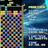## Counting Bejeweled Boards

I’ve recently become hooked on Bejeweled Blitz, an addictive match-three puzzle game. It’s got enough bright colors, explosive sounds, and variable reward mechanics to redline your dopamine levels.

Coders have an interesting coping mechanism to deal with these sorts of things: program a bot to automate the addiction. This is a bit better than grinding for points; it’s a nice programming exercise that is slightly subversive and without intellectual property issues that could worry one’s employer.

During the design phase of the bot, I’ve become stuck on a little mathematical question that has led me on a journey from coding to mathematics via some impressively knowledge-dense internet resources:

How many different Bejeweled boards are there?

### Problem Statement

Match-three tile games like Bejeweled are played on a square grid with colored tiles. The game begins with a randomly generated board that satisifies the following conditions:

• No horizontal or vertical three-in-a-row runs of the same color
• At least one pair of neighboring colors can be swapped to create a horizontal or vertical three-in-a-row run

The figure below shows a legal 4-by-4 board on the left and an illegal starting board on the right.Bejeweled is played on an 8-by-8 board using tiles of 7 colors at the start, so an upper bound on the number of initial legal boards is$7^{8 \times 8}$. This is about the number of atoms in ten thousand Earth-sized planets!

### Color Normalization

The colors of the tiles on the board do not actually define a unique board position, according to the rules of the game. Consider the figure below; the second board is a copy of the first board with its tile colors permuted.The first two boards are identical with respect to which actions can be taken.

To avoid counting boards with permuted colors more than once, one can define a mapping that normalizes the colors on any proposed board, and then refuse to count the same color normalized board more than once. The normalization scheme used in this post assigns each color a number according to the order in which they occur on the board, from left-to-right and top-to-bottom. This is what the third board in the figure represents– notice how it captures the game-relevant information in both of the first two boards.

This representation is somewhat smaller than the original upper bound of$7^{64}$. As a trivial proof of this, note that there is now only one possible color value for the upper-left tile instead of seven.

### Brute-Force Count

Perhaps a computer program could count the number of unique normalized boards? This JSFiddle is a Javscript program that enumerates all normalized tile sequences of a certain length. Notice how quickly the numbers grow from a board with 1 tile to a board with 13 tiles: 1, 2, 5, 15, 52, 203, 877, 4139, 21110, 115179, 665479, 4030523, 25343488. There is no way that this approach will scale to 64 tiles.

### Online Encyclopedia of Integer Sequences

Faced with this computational hurdle, it is expedient to employ the primary tool of the curious modern slacker: internet search. A query to the Online Encyclopedia of Integer Sequences (OEIS) finds the sequence A099262. Turns out our sequence-of-interest shares the same starting entries as the partial sum of Stirling Numbers of the Second Kind:$\sum_{k{=}1}^c S(n,k) = \sum_{k{=}1}^c \left[ \frac{1}{k!} \sum_{j{=}0}^k\left(-1\right)^{k-j}\binom{k}{j}j^n \right]$

Where$c=7$ and$n=64$ for the standard Bejeweled Blitz board. This is an easy equation to solve with the right tools, but it remains to be shown that the two sequences are actually identical.

### Stirling Numbers of the Second Kind

Stirling Numbers of the Second Kind, written as$S(n,k)$, are the number of ways to partition a set of$n$ labeled objects into$k$ non-empty, unlabeled subsets. Below, we relate the things that this sequence is counting with the unique, color-normalized board instances.

Let each of the 64 tiles on the board be one of the$n$ labeled objects. Let the total number of different colors on the color-normalized board be the value of$k$. For the moment, let’s count only the number of color-normalized boards containing exactly$k$ different colors. The quantity$S(n, k)$ is the number of ways one can assign each tile to one of the color-related subsets.

The trick now is to show that there is a way to label the unlabeled subsets counted by$S(n,k)$ such that the labels respect our normalization scheme. First, take the subset containing the first tile on the board and assign it the label “color 1”. Then, find the next tile on the board that is not in this set and assign it the label “color #2”. Continue until all subsets have been assigned color labels. Since$S(n,k)$ counts only non-empty subsets, each of the$k$ colors will be labeled with a color number.

This should be a good start to a convincing explanation, although there are missing details that a real proof requires. The remaining work involves adding up the counts for all initial boards with only one color, only two colors, and so on up to$k$ colors. This is where the outer-most sum comes into play.

### Conclusion

A WolframAlpha query Sum[StirlingS2[64,i],{i,1,7}] in scientific notation calculates the exact number of color-normalized boards. This rounds to$2.42 \times 10^{50}$, which is still an enormous number that is slightly greater than the estimated number of atoms in Earth. But it is four orders of magnitude smaller than our original upper-bound!

I hope to find the time to continue to chip away at this upper-bound by finding ways to throw away the color-normalized boards that don’t respect the match-three constraints. For the moment, I’m content to marvel at the crazy path from programming, through internet search, through actual mathematics, to winding up at a solution that can be evaluated in seconds in an accessible mathematical tool.

1.Farter Yang (@farteryhr) Says: